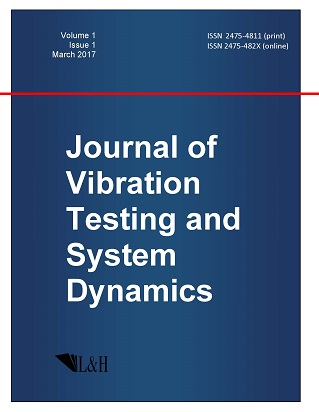ISSN: 2475-4811 (print)
ISSN: 2475-482X (online)
Journal of Vibration Testing and System Dynamics

C. Steve Suh (editor), Pawel Olejnik (editor),

Xianguo Tuo (editor)

Pawel Olejnik (editor)

Lodz University of Technology, Poland

Email: pawel.olejnik@p.lodz.pl

C. Steve Suh (editor)

Texas A&M University, USA

Email: ssuh@tamu.edu

Xiangguo Tuo (editor)

Sichuan University of Science and Engineering, China

Email: tuoxianguo@suse.edu.cn

Higher Order First Integrals of Autonomous Dynamical Systems in Terms of Geometric Symmetries

Journal of Vibration Testing and System Dynamics 7(1) (2023) 23--30 | DOI:10.5890/JVTSD.2023.03.004

Antonios Mitsopoulos, Michael Tsamparlis

Faculty of Physics, Department of Astronomy-Astrophysics-Mechanics, University of Athens, Panepistemiopolis, Athens 157 83, Greece

Abstract

In general, a system of differential equations is integrable if there exist sufficiently many' first integrals (FIs) so that its solution can be found by means of quadratures. Therefore, the determination of the FIs is an important issue in order to establish the integrability of a dynamical system. In this work, we consider holonomic autonomous dynamical systems defined by equations $\ddot{q}^{a}= -\Gamma_{bc}^{a}(q) \dot{q}^{b}\dot{q}^{c} -Q^{a}(q)$ where $\Gamma^{a}_{bc}(q)$ are the coefficients of a symmetric (possibly non-metrical) connection and $-Q^{a}(q)$ are the generalized forces. We prove a theorem which produces the FIs of any order of such systems in terms of the symmetries' of the geometry defined by the quantities $\Gamma_{bc}^{a}(q)$. We apply the theorem to compute quadratic and cubic FIs of various dynamical systems.

References

1.   Arnold, V.I. (1989), Mathematical Methods of Classical Mechanics, Springer, 272-284.
2.   Damianou, P.A. and Sophocleous, C. (2004), Classification of Noether symmetries for Lagrangians with three degrees of freedom, Nonlinear Dynamics, 36, 3-18.
3.   Tsamparlis, M. and Paliathanasis, A. (2011), Two-dimensional dynamical systems which admit Lie and Noether symmetries, Journal of Physics A: Mathematical and Theoretical, 44, 175202.
4.   Halder, A.K., Paliathanasis, A., and Leach, P.G.L. (2018), Noether's theorem and symmetry, Symmetry, 10(12), 744.
5.   Katzin, G.H. and Levine, J. (1981), Geodesic first integrals with explicit path-parameter dependence in Riemannian space-times, Journal of Mathematical Physics, 22(9), 1878-1891.
6.   Horwood, J.T. (2007), Higher order first integrals in classical mechanics, Journal of Mathematical Physics, 48, 102902.
7.   Tsamparlis, M. and Mitsopoulos, A. (2020), Quadratic first integrals of autonomous conservative dynamical systems, Journal of Mathematical Physics, 61, 072703.
8.   Tsamparlis, M. and Mitsopoulos, A. (2020), First integrals of holonomic systems without Noether symmetries, Journal of Mathematical Physics, 61, 122701.
9.   Mitsopoulos, A. and Tsamparlis, M. (2021), Higher order first integrals of autonomous dynamical systems, Journal of Geometry and Physics, 170, 104383.
10.   Djukic, D.S. and Vujanovic, B.D. (1975), Noether's theory in classical nonconservative mechanics, Acta Mechanica, 23, 17-27.
11.   Fokas, A.S. and Lagerstrom, P.A. (1980), Quadratic and cubic invariants in classical mechanics, Journal of Mathematical Analysis and Applications, 74, 325-341.
12.   Karlovini, M. and Rosquist, K. (2000), A unified treatment of cubic invariants at fixed and arbitrary energy, Journal of Mathematical Physics, 41(1), 370-384.